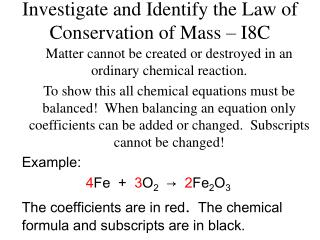DownloadDownload PresentationInvestigate and Identify the Law of Conservation of Mass – I8C

# Investigate and Identify the Law of Conservation of Mass – I8C

Download Presentation## Investigate and Identify the Law of Conservation of Mass – I8C

- - - - - - - - - - - - - - - - - - - - - - - - - - - E N D - - - - - - - - - - - - - - - - - - - - - - - - - - -
##### Presentation Transcript

1. Investigate and Identify the Law of Conservation of Mass – I8C Matter cannot be created or destroyed in an ordinary chemical reaction. To show this all chemical equations must be balanced! When balancing an equation only coefficients can be added or changed. Subscripts cannot be changed! Example: 4Fe + 3O2→ 2Fe2O3 The coefficients are in red. The chemical formula and subscripts are in black.

2. To balance an equation take an “atom inventory” on both sides of the equation: ___K + ___H2O  ___KOH + ___H2 Reactants Products K: 1 K: 1 H: 2 H: 3 O: 1 O: 1

3. Next choose coefficients to balance the equation: _2_K + _2_H2O  _2_KOH + _1_H2 Reactants Products K: 1 2 K: 1 2 H: 2 4 H: 3 4 O: 1 2 O: 1 2

4. Practice Problems: (All practice problems are from TEA released TAKS Tests) 1. What are the coefficients that will balance this chemical equation? A 2, 1, 1 B 3, 4, 2 C 2, 2, 1 D 4, 3, 2

5. 2. The chemical equation shows CaCO3 being heated. Which of these statements best describes the mass of the products if 100 g of CaCO3 is heated? A The difference in the products’ masses is equal to the mass of the CaCO3. B The sum of the products’ masses is less than the mass of the CaCO3. C The mass of each product is equal to the mass of the CaCO3. D The sum of the products’ masses equals the mass of the CaCO3. (All practice problems are from TEA released TAKS Tests)

6. 3. Which chemical equation supports the law of conservation of mass? F 2H2O(l) → H2(g) + O2(g) G Zn(s) + HCl(aq) → ZnCl2(aq) + H2(g) H Al4C3(s) + H2O(l) → CH4(g) + Al(OH)3(s) J CH4(g) + 2O2(g) → CO2(g) + 2H2O(g) (All practice problems are from TEA released TAKS Tests)

7. 4. When 127 g of copper reacts with 32 g of oxygen gas to form copper (II) oxide, no copper or oxygen is left over. How much copper (II) oxide is produced? F 32 g G 95 g H 127 g J 159 g (All practice problems are from TEA released TAKS Tests)

8. 5. When the above equation is balanced, the coefficient for magnesium chloride is — A 0 B 1 C 2 D 4 (All practice problems are from TEA released TAKS Tests)

9. 6. According to the law of conservation of mass, how much zinc was present in the zinc carbonate? A 40 g B 88 g C 104 g D 256 g (All practice problems are from TEA released TAKS Tests)

10. 7. According to this information, what is the chemical formula for aluminum sulfate? A AlSO4 B Al2(SO4)3 C Al3(SO4)2 D Al6SO4 (All practice problems are from TEA released TAKS Tests)

11. 8. The chemical formula for calcium chloride is — F Ca2Cl G CaCl H CaCl2 J Ca2Cl3 (All practice problems are from TEA released TAKS Tests)

12. In the procedure shown above, a calcium chloride solution is mixed with a sodium sulfate solution to create the products shown. Which of the following is illustrated by this activity?(A) The law of conservation of mass (B) The theory of thermal equilibrium(C) The law of conservation of momentum (D) The theory of covalent bonding

13. __Ca(C2H3O2)2 + __K3PO4 → __Ca3(PO4)2 + __KC2H3O2 10. Which set of coefficients balances the equation above? (F) 3, 3, 1, 2 (G) 6, 1, 1, 3 (H) 3, 2, 1, 6 (J) 6, 2, 1, 6